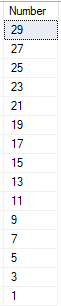# How to use SQL Union in Set Operations

In this puzzle, we’re going to learn how to write a SQL UNION without using Set Operations.  Set operations allow us to compare rows from two or more tables to arrive at a result.   For several classes of problems, it is much easier to use a set operation, than join data.  But can we do without a SQL UNION?

Yet a great way to know and understand SQL is to explore alternative methods.  Today we’ll chuck the SQL UNION operator out the door, and seek an alternative method to arrive at the same result.

Are you up to the challenge?

Solving puzzles is a great way to learn SQL.  Nothing beats practicing what you’ve learned.  Once you have figured out the puzzle, post you answer in the comments so we all can learn from one another.  We also discuss puzzle and more in Essential SQL Learning Group on Facebook.  Be sure to find us there!

## SQL Union Two Results Using No UNION

Given two lists of numbers: A and B

Select all odd numbers ordered from highest to lowest into a single result using one statement WITHOUT Using any SET Operators such as UNION, EXCEPT, or INTERSECT.

Here is a script you can use to create the tables.

Here is the solution I came up with today:

```WITH cteNumber (Number)
AS
(
SELECT ISNULL(A.Number, B.Number)
FROM   @A A
FULL OUTER JOIN @B B
ON A.Number = B.Number
)
SELECT   Number
FROM     cteNumber
WHERE    Number % 2 = 1
ORDER BY Number DESC```

There are several elements I’ll go over; however, let’s start with the one  that really makes this tick – the FULL OUTER JOIN.

A FULL OUTER JOIN combines a LEFT and RIGHT OUTER JOIN into one operation.  This allow us to return every row from both tables.  Where the join condition isn’t met, NULLs are returned.

Here is an example:

```SELECT A.Number ANumber, B.Number BNumber
FROM   @A A
FULL OUTER JOIN @B B
ON A.Number = B.Number```

And the results shown rows exclusive to their respective table, as well as those in common.If you look closely, you’ll see that we have the beginnings of an UNION operation.  All that we need to do is “combine” the ANumber and BNumber column values together for each row.

Do to this we use the ISNULL function.  The function ISNULL(value, replacementValue) returns a value, and if value IS NULL, then replacementValue.

Thus,

`ISNULL(ANumber, BNumber)`

returns ANumber, and if ANumber IS NULL, then BNumber.

Given this, we can modify our query to:

```SELECT ISNULL(A.Number, B.Number)
FROM   @A A
FULL OUTER JOIN @B B
ON A.Number = B.Number```

Which now returns the union of Number for tables A and B.

To finish the solution, I wrapped this FULL OUTER JOIN in a CTE (Common Table Expression).  Then, queried the results, checked for odd numbers, and sorted in descending order.

```WITH cteNumber (Number)
AS
(
SELECT ISNULL(A.Number, B.Number)
FROM   @A A
FULL OUTER JOIN @B B
ON A.Number = B.Number
)
SELECT   Number
FROM     cteNumber
WHERE    Number % 2 = 1
ORDER BY Number DESC```

Keep in mind that % operator returns the modulo (remainder) of a division.  Any number when divided by 2 whose remainder is 1, is odd.

Also, to sort number from highest to lowest, use the DESC (descending) qualifier.

Here are the results:## Alternative Solution

If you don’t like common table expressions, or your version of SQL doesn’t support them, then you’ll find this answer a bit more traditional:

```SELECT ISNULL(A.Number, B.Number) as Number
FROM   @A A
FULL OUTER JOIN @B B
ON A.Number = B.Number
WHERE  A.Number % 2 = 1 OR
B.Number % 2 = 1
ORDER BY Number```

If you’re like me, you’re most likely looking at the WHERE clause and wondering why we’re using OR rather than AND.

At first I thought AND would be the way to go, after all we only want to keep odd values from both tables.  Yet, once I thought about it, I realized some of the columns return NULL, and NULL % 2 is NULL, so using AND would limit any rows exclusive to one table.

I then thought about OR.  With this Boolean operator, we’re ensuring we get the odd values, even if the other columns is NULL, and in the case both columns aren’t null, they are equal, due to the join operator, so OR in this case is OK!

#### Kris Wenzel

Kris Wenzel has been working with databases over the past 30 years as a developer, analyst, and DBA. He has a BSE in Computer Engineering from the University of Michigan and a MBA from the University of Notre Dame. Kris has written hundreds of blog articles and many online courses. He loves helping others learn SQL.